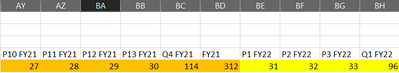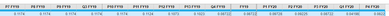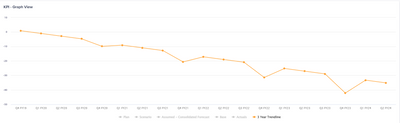# Filer Q and Year, to Calculate line itemsedited December 2022 in Modeling

Hi All,

My issue is that I'm using a graph to show the P&L, but my 3-year trendline is spiking, when set to Q and Years.

So I have P&L line items calculations and they are connected to different module views, so I created time filters modules to calculate the Periodic, Yearly, and Quarters.

So, if I change in the line items the summary mode to calculate yearly and quarterly values to formula, average it affects my other grids.

So is there a way I can set the quarterly view with this characteristic, the time calendar is set to 3-3-3-4

In my time module I have set a time diff line item so it can take the periods through a 3-year trend, the problem is that when I calculate Q or years the calculated number is too big because is taking for Q4 - 4 periodsSo is there a way I can alter through the filters the way it calculates my data.

Thanks!

Tagged:

•edited December 2022

Hi @Diepcc

Can you please provide an example of the calculation that you are trying to do in monthly levels and then what is expected in quarterly/ yearly numbers?

•Hi @anikdas

For example here I have the organic growth calculation.As you can see my model is set to be 3-3-3-4, which is why Q4 is always bigger than next year Q1.

So my graph gets this spikes when getting to Q4So what I'm asking for help is if there is a way to solve this, without changing all my P&L lines to, formula-average, since this impacts my periodic figures

Thanks for taking the time